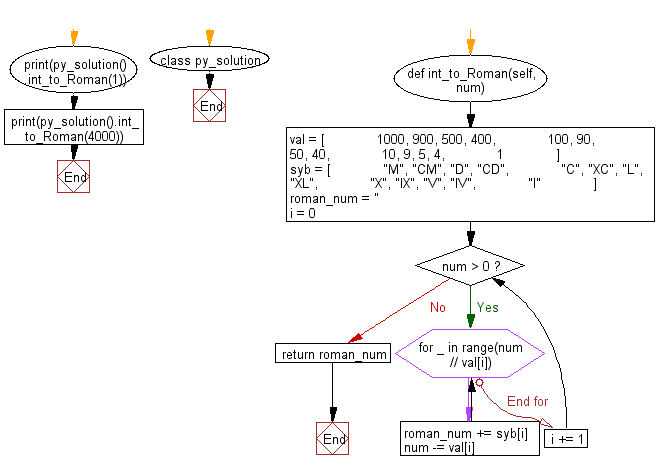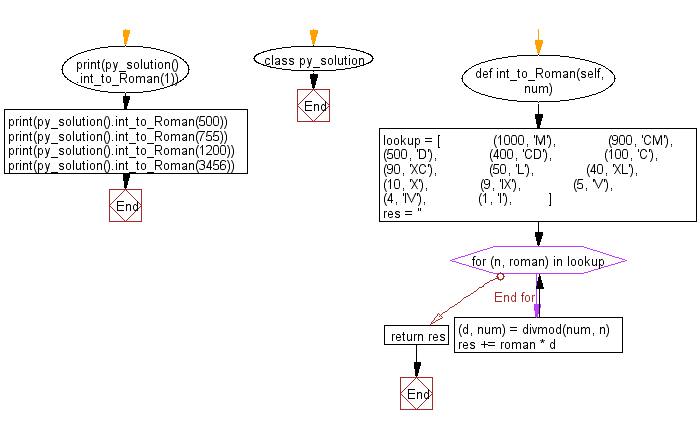﻿ Python: Convert an integer to a roman numeral - w3resource# Python: Convert an integer to a roman numeral

## Python Class: Exercise-1 with Solution

Write a Python class to convert an integer to a roman numeral.

Sample Solution-1:

Python Code:

``````class py_solution:
def int_to_Roman(self, num):
val = [
1000, 900, 500, 400,
100, 90, 50, 40,
10, 9, 5, 4,
1
]
syb = [
"M", "CM", "D", "CD",
"C", "XC", "L", "XL",
"X", "IX", "V", "IV",
"I"
]
roman_num = ''
i = 0
while  num > 0:
for _ in range(num // val[i]):
roman_num += syb[i]
num -= val[i]
i += 1
return roman_num

print(py_solution().int_to_Roman(1))
print(py_solution().int_to_Roman(4000))
```
```

Sample Output:

```I
MMMM
```

Pictorial Presentation:Flowchart:## Visualize Python code execution:

The following tool visualize what the computer is doing step-by-step as it executes the said program:

Sample Solution-2:

Convert an integer to its roman numeral representation. Accepts value between 1 and 3999 (both inclusive).

• Create a lookup list containing tuples in the form of (roman value, integer).
• Use a for loop to iterate over the values in lookup.
• Use divmod() to update num with the remainder, adding the roman numeral representation to the result.

Python Code:

``````class py_solution:
def int_to_Roman(self, num):
lookup = [
(1000, 'M'),
(900, 'CM'),
(500, 'D'),
(400, 'CD'),
(100, 'C'),
(90, 'XC'),
(50, 'L'),
(40, 'XL'),
(10, 'X'),
(9, 'IX'),
(5, 'V'),
(4, 'IV'),
(1, 'I'),
]
res = ''
for (n, roman) in lookup:
(d, num) = divmod(num, n)
res += roman * d
return res
print(py_solution().int_to_Roman(1))
print(py_solution().int_to_Roman(500))
print(py_solution().int_to_Roman(755))
print(py_solution().int_to_Roman(1200))
print(py_solution().int_to_Roman(3456))
```
```

Sample Output:

```I
D
DCCLV
MCC
MMMCDLVI
```

Flowchart:## Visualize Python code execution:

The following tool visualize what the computer is doing step-by-step as it executes the said program:

Python Code Editor:

What is the difficulty level of this exercise?

Test your Programming skills with w3resource's quiz.

﻿

## Python: Tips of the Day

The Zip() Function:

```>>> students = ('John', 'Mary', 'Mike')
>>> ages = (15, 17, 16)
>>> scores = (90, 88, 82, 17, 14)
>>> for student, age, score in zip(students, ages, scores):
...     print(f'{student}, age: {age}, score: {score}')
...
John, age: 15, score: 90
Mary, age: 17, score: 88
Mike, age: 16, score: 82
>>> zipped = zip(students, ages, scores)
>>> a, b, c = zip(*zipped)
>>> print(b)
(15, 17, 16)
```